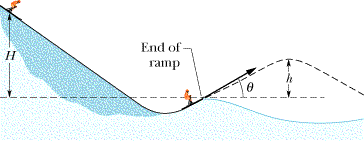# Conservation of Energy ski jump problemA 78 kg skier starts from rest at a height of H = 29 m above the end of the ski-jump ramp (Figure 8-39). As the skier leaves the ramp, his velocity makes an angle of 28° with the horizontal. Neglect the effects of air resistance and assume the ramp is frictionless.
(a) What is the maximum height h of his jump above the end of the ramp?
____ m

m = 60 kg
H = 20 m
theta = 28
h = ?

I used the equation

Ki + Ui = Kf + Uf
(Initial/Final Kinetic + Potential Energy)
1/2mVi^2 + mgyi = 1/2mVf^2 + mgyf
1/2(0)^2 + (9.8)(20) = 1/2m(Vf)^2 + gh

But I dont know how to get Vf. Where do I use theta?

jamesrc
Gold Member
The problem must be done in two parts. Use the conservation of energy equation you have written to find vf which is the speed of the jumper at the time he leaves the ramp. H=29m according to your problem statement, not 20 m. And yf=0, not h, because "f" refers to the time when the jumper is at the end of the ramp and the height he is at at that point (when his speed is vf) is 0. Once you have found vf, you use the angle to find the x and y components of the velocity. This can then be used with either kinematic equations or more energy conservation equations to find the max height h.

daniel_i_l
Gold Member
Or you could find the horizontal speed at the highest point of his trajectory after he leaves the ramp and use energy conservation to find the height.

i got Vf = 23.84

Do I plug in the y-component of Vf?

daniel_i_l
Gold Member
The Vf should be the speed at the highest point of the trajectory after going off the ramp, it only has a x component.

jamesrc
Gold Member
i got Vf = 23.84

Do I plug in the y-component of Vf?

What are your units? You plugged in your numbers properly and got the right answer, but your answer is incomplete without units. I don't mean to be too pedantic, but if I were grading you, I'd take off for that.

Anyway, to finish solving the problem...

yes you need to find the y-component of vf. That speed is directed at an angle of whatever they told you &theta; was. In order to find his maximum height, you need to find consider the vertical component of that velocity.

i got Vf = 23.84

Do I plug in the y-component of Vf?

Your value of Vf is incorrect, probably because you left the term 'm' in your equation. Also, 'gh' shouldn't be there in the RHS, because the diagram clearly shows them at the same level.

Using mgh = 1/2 m v^2 (because initially, it has only potential energy, before going off ramp, only kinetic energy), you get
v = (2gh)^(1/2) = 20 m/s (approx).

Now, using this in equation for maximum height attained for projectile motion:
Max height = ((v sin theta)^2)/(2g)

The above can be derived from the parametric equation of projectile motion. Plug the values in, and you get max height = ((20 x sin 28 degree)^2)/(2 x 9.8)) = 4.45 m.

You can even analyze the skier as a projectile. The velocity at max ht is u*cos$$\theta$$. thus the kinetic energy at max height is
K.E= 0.5m*(ucos$$\theta$$)^2
You can use this in the conservation of energy eqn you have written...